# RS Aggarwal Solutions for Class 8 Chapter 1 - Rational Numbers Exercise 1H

RS Aggarwal Solutions for Class 8 Maths Chapter 1, Rational Numbers contains solutions to the questions related to Exercise 1H, downloaded from the links provided below. Hereby students can practice the RS Aggarwal Solutions to ensure they are thorough with their fundamentals.

In Exercise 1H of RS Aggarwal Class 8 Maths, we shall brief all the concepts covered in this Chapter 1 i.e. Positive and Negative rational numbers, Representation of Rational Numbers on the Number line, Addition, Subtraction, Multiplication and Division of Rational Numbers using their properties.

## Download PDF of RS Aggarwal Solutions for Class 8 Maths Chapter 1- Exercise 1H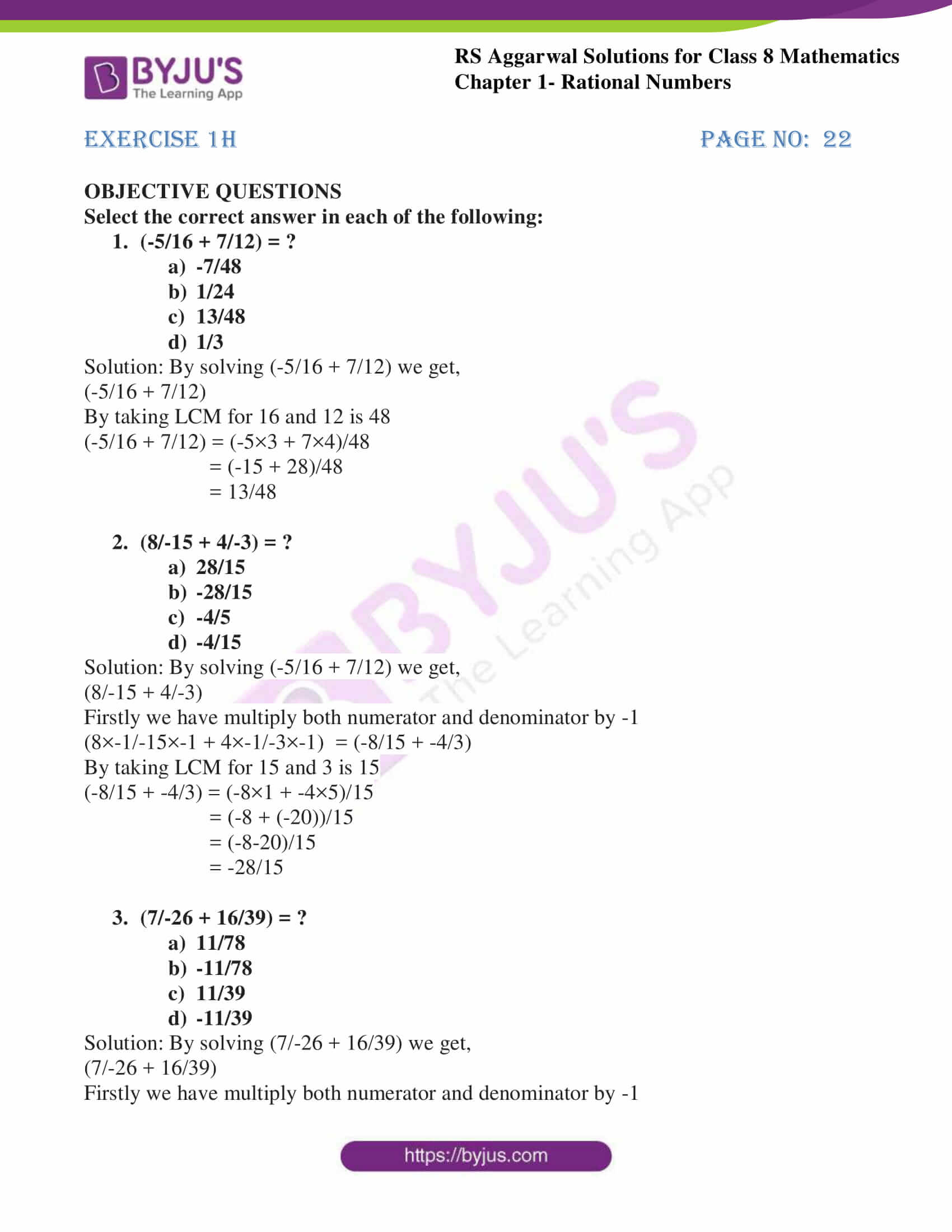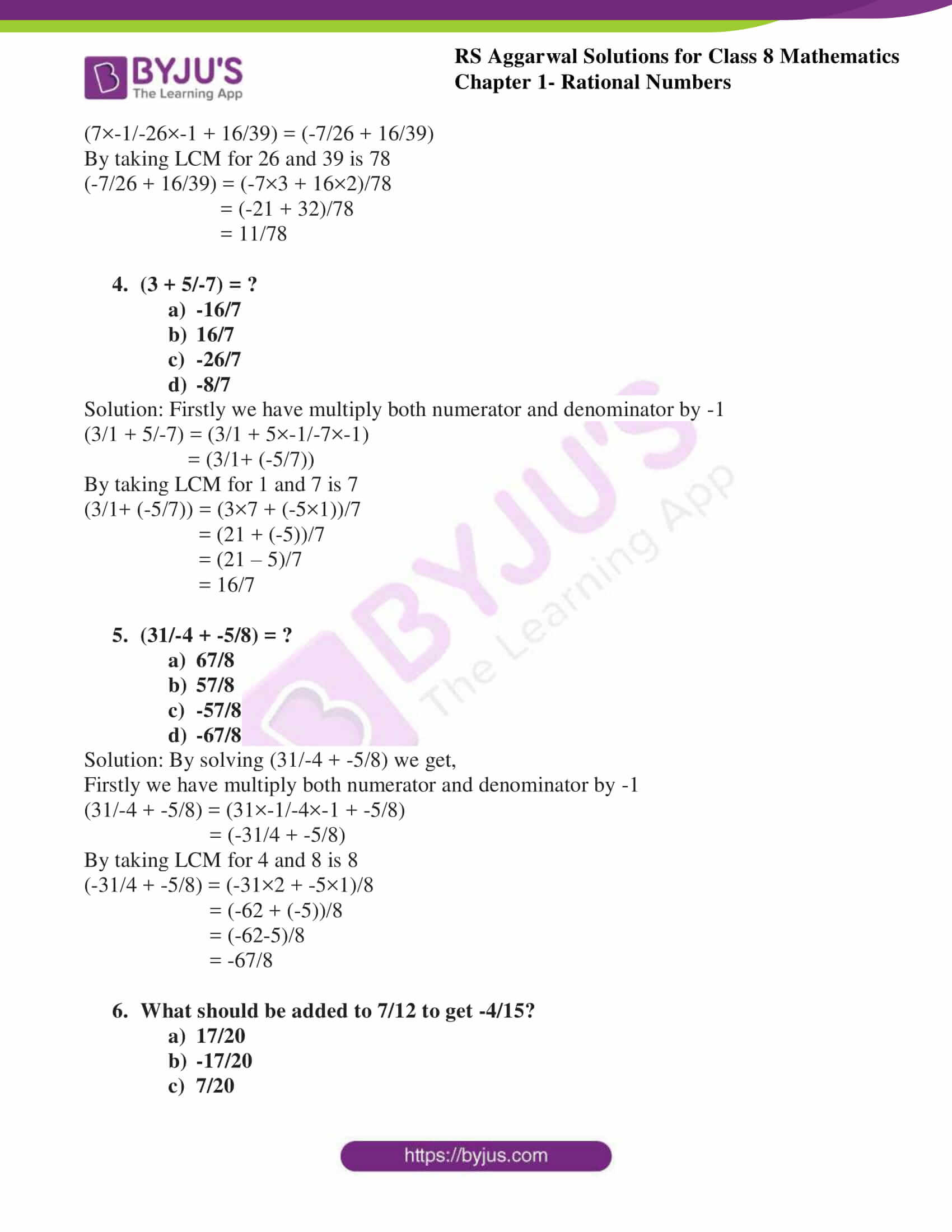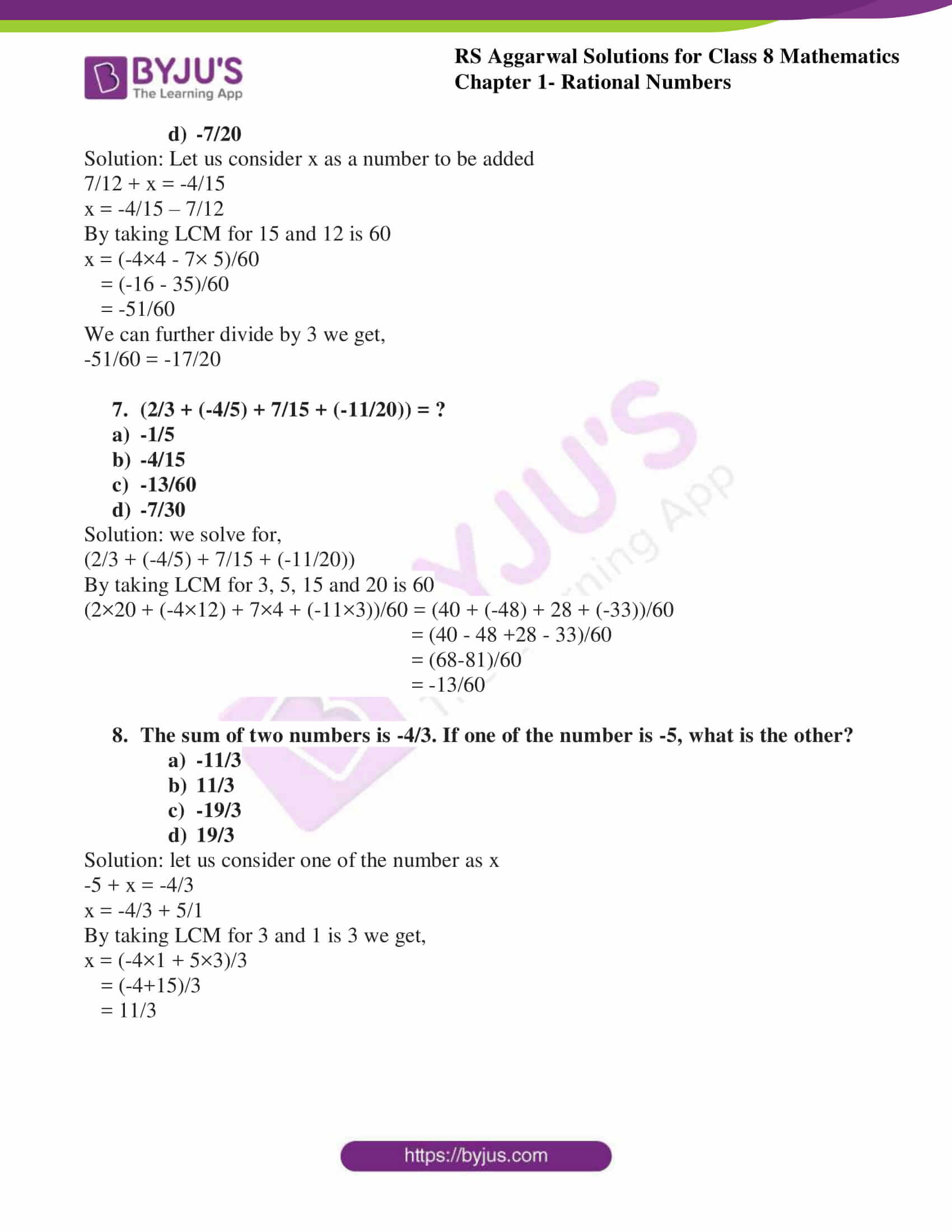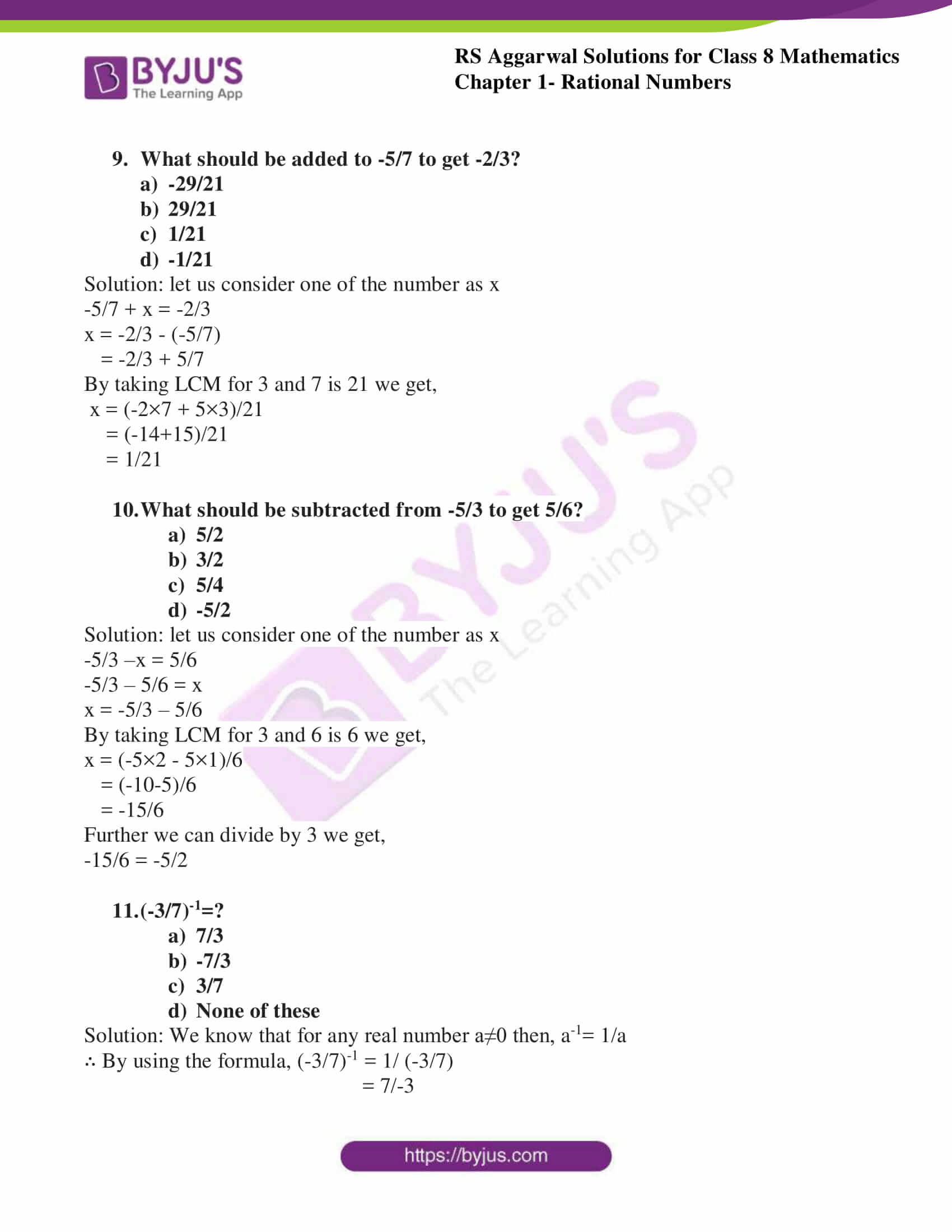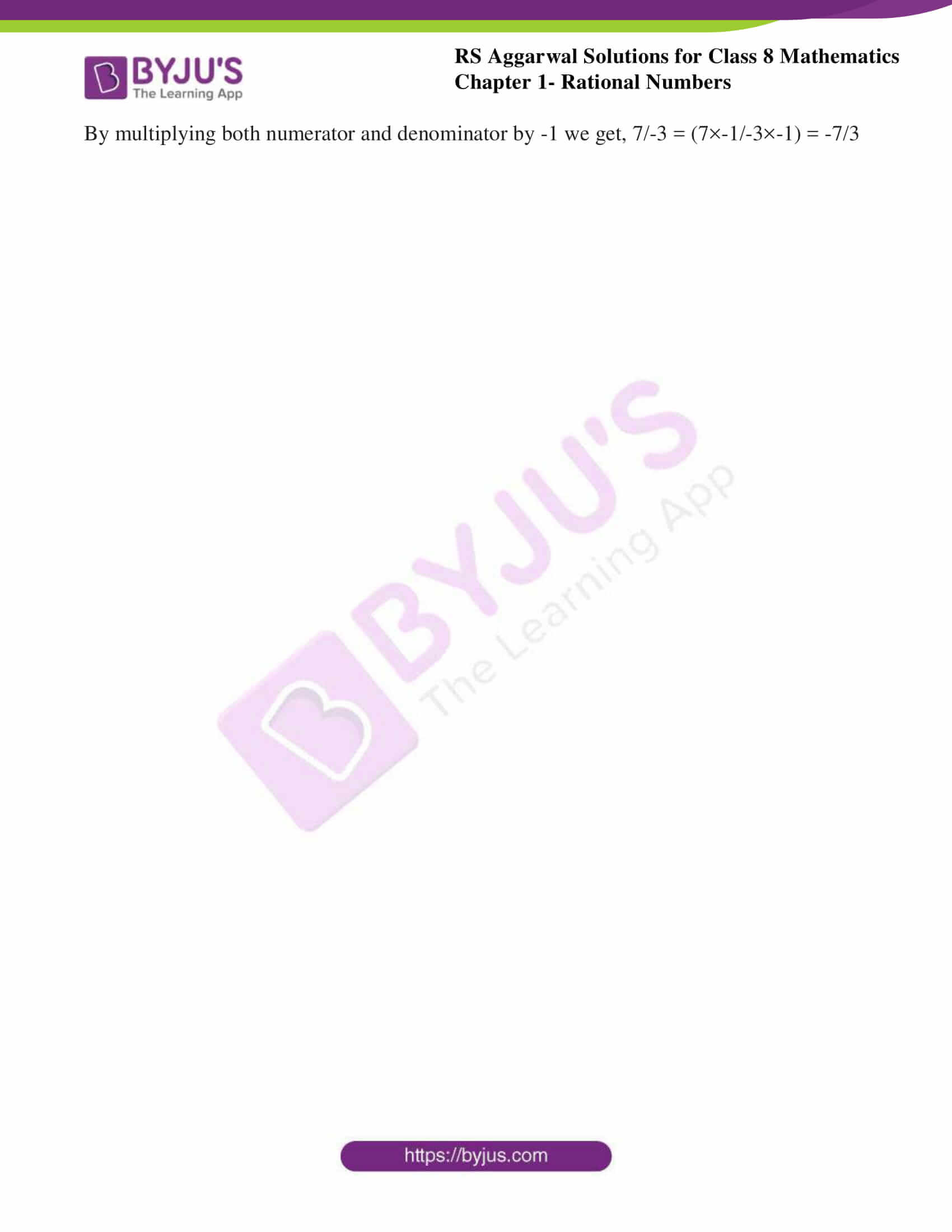### Access answers to RS Aggarwal Solutions for Class 8 Maths Chapter 1- Rational Numbers Exercise 1H

Select the correct answer in each of the following:

1. (-5/16 + 7/12) = ?

1. -7/48
2. 1/24
3. 13/48
4. 1/3

Solution: By solving (-5/16 + 7/12) we get,(-5/16 + 7/12)

By taking LCM for 16 and 12 is 48

(-5/16 + 7/12) = (-5×3 + 7×4)/48

= (-15 + 28)/48

= 13/48

2. (8/-15 + 4/-3) = ?

1. 28/15
2. -28/15
3. -4/5
4. -4/15

Solution: By solving (-5/16 + 7/12) we get,(8/-15 + 4/-3)

Firstly we have multiply both numerator and denominator by -1

(8×-1/-15×-1 + 4×-1/-3×-1) = (-8/15 + -4/3)

By taking LCM for 15 and 3 is 15

(-8/15 + -4/3) = (-8×1 + -4×5)/15

= (-8 + (-20))/15

= (-8-20)/15

= -28/15

3. (7/-26 + 16/39) = ?

1. 11/78
2. -11/78
3. 11/39
4. -11/39

Solution: By solving (7/-26 + 16/39) we get,(7/-26 + 16/39)

Firstly we have multiply both numerator and denominator by -1

(7×-1/-26×-1 + 16/39) = (-7/26 + 16/39)

By taking LCM for 26 and 39 is 78

(-7/26 + 16/39) = (-7×3 + 16×2)/78

= (-21 + 32)/78

= 11/78

4. (3 + 5/-7) = ?

1. -16/7
2. 16/7
3. -26/7
4. -8/7

Solution: Firstly we have multiply both numerator and denominator by -1(3/1 + 5/-7) = (3/1 + 5×-1/-7×-1)

= (3/1+ (-5/7))

By taking LCM for 1 and 7 is 7

(3/1+ (-5/7)) = (3×7 + (-5×1))/7

= (21 + (-5))/7

= (21 – 5)/7

= 16/7

5. (31/-4 + -5/8) = ?

1. 67/8
2. 57/8
3. -57/8
4. -67/8

Solution: By solving (31/-4 + -5/8) we get,Firstly we have multiply both numerator and denominator by -1

(31/-4 + -5/8) = (31×-1/-4×-1 + -5/8)

= (-31/4 + -5/8)

By taking LCM for 4 and 8 is 8

(-31/4 + -5/8) = (-31×2 + -5×1)/8

= (-62 + (-5))/8

= (-62-5)/8

= -67/8

6. What should be added to 7/12 to get -4/15?

1. 17/20
2. -17/20
3. 7/20
4. -7/20

Solution: Let us consider x as a number to be added7/12 + x = -4/15

x = -4/15 – 7/12

By taking LCM for 15 and 12 is 60

x = (-4×4 – 7× 5)/60

= (-16 – 35)/60

= -51/60

We can further divide by 3 we get,

-51/60 = -17/20

7. (2/3 + (-4/5) + 7/15 + (-11/20)) = ?

1. -1/5
2. -4/15
3. -13/60
4. -7/30

Solution: we solve for,(2/3 + (-4/5) + 7/15 + (-11/20))

By taking LCM for 3, 5, 15 and 20 is 60

(2×20 + (-4×12) + 7×4 + (-11×3))/60 = (40 + (-48) + 28 + (-33))/60

= (40 – 48 +28 – 33)/60

= (68-81)/60

= -13/60

8. The sum of two numbers is -4/3. If one of the number is -5, what is the other?

1. -11/3
2. 11/3
3. -19/3
4. 19/3

Solution: let us consider one of the number as x-5 + x = -4/3

x = -4/3 + 5/1

By taking LCM for 3 and 1 is 3 we get,

x = (-4×1 + 5×3)/3

= (-4+15)/3

= 11/3

9. What should be added to -5/7 to get -2/3?

1. -29/21
2. 29/21
3. 1/21
4. -1/21

Solution: let us consider one of the number as x-5/7 + x = -2/3

x = -2/3 – (-5/7)

= -2/3 + 5/7

By taking LCM for 3 and 7 is 21 we get,

x = (-2×7 + 5×3)/21

= (-14+15)/21

= 1/21

10. What should be subtracted from -5/3 to get 5/6?

1. 5/2
2. 3/2
3. 5/4
4. -5/2

Solution: let us consider one of the number as x-5/3 –x = 5/6

-5/3 – 5/6 = x

x = -5/3 – 5/6

By taking LCM for 3 and 6 is 6 we get,

x = (-5×2 – 5×1)/6

= (-10-5)/6

= -15/6

Further we can divide by 3 we get,

-15/6 = -5/2

11. (-3/7)-1=?

1. 7/3
2. -7/3
3. 3/7
4. None of these

Solution: We know that for any real number a≠0 then, a-1= 1/a

∴ By using the formula, (-3/7)-1 = 1/ (-3/7)

= 7/-3

By multiplying both numerator and denominator by -1 we get, 7/-3 = (7×-1/-3×-1) = -7/3

## RS Aggarwal Solutions for Class 8 Maths Chapter 1- Exercise 1H

Exercise 1H of RS Aggarwal Class 8 Chapter 1, Rational Numbers contains the advance concepts related to Rational Numbers. This exercise mainly deals with Objective Questions related to the entire Chapter 1.

The RS Aggarwal Solutions can help the students practice and learn the fundamentals as it provides all the answers to the questions from the RS Aggarwal textbook. Practicing as many times as possible helps in a better understanding of the concept and also helps in effective time management skills. It also boosts the confidence level to achieve high marks.